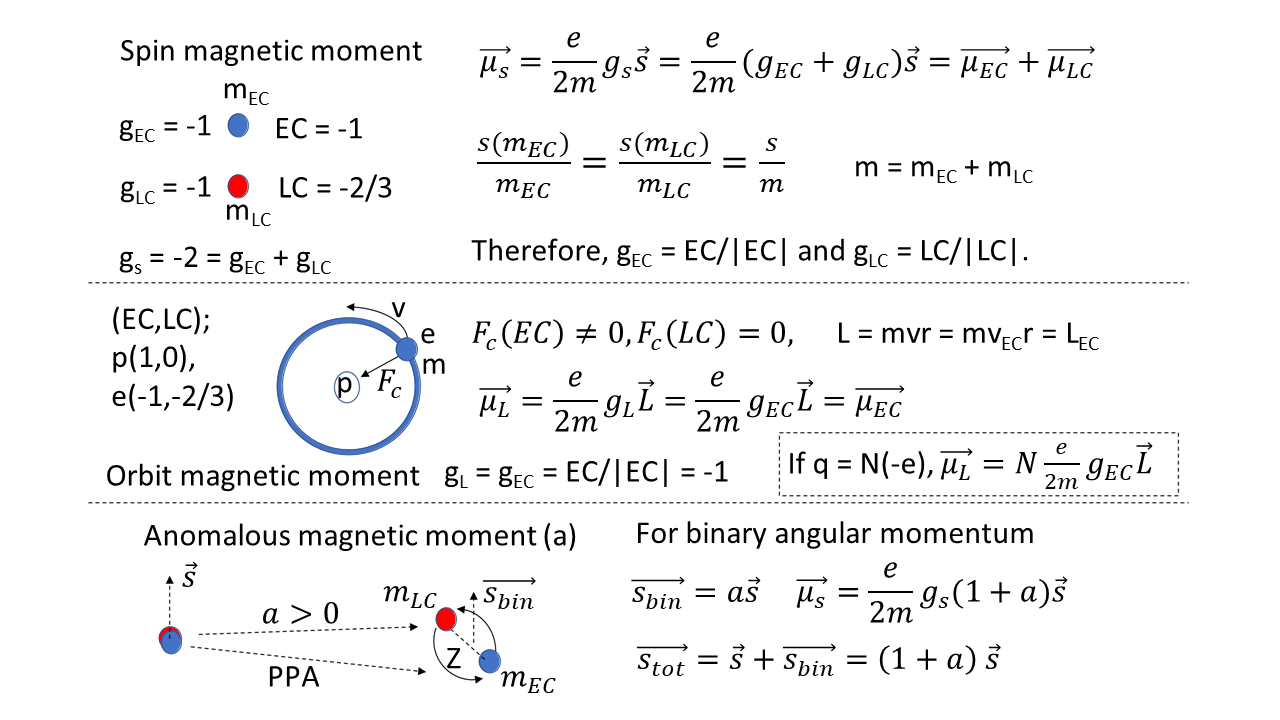Preprint Article Version 1 Preserved in Portico This version is not peer-reviewed

# Spin g Factors and Neutrino Magnetic Moment of Elementary Fermions

Version 1 : Received: 20 December 2021 / Approved: 21 December 2021 / Online: 21 December 2021 (13:49:29 CET)
Version 2 : Received: 28 February 2022 / Approved: 1 March 2022 / Online: 1 March 2022 (11:25:10 CET)

How to cite: Hwang, J. Spin g Factors and Neutrino Magnetic Moment of Elementary Fermions. Preprints 2021, 2021120340 (doi: 10.20944/preprints202112.0340.v1). Hwang, J. Spin g Factors and Neutrino Magnetic Moment of Elementary Fermions. Preprints 2021, 2021120340 (doi: 10.20944/preprints202112.0340.v1).

## Abstract

The spin magnetic moments and spin g factors (gs = -2) of electron, muon and tau are explained based on the electric charges (EC) and lepton charges (LC) in terms of the three-dimensional quantized space model. The spin g factors of electron, muon and tau are gs = -2 which is the sum of the EC g factor (gEC = -1) and the LC g factor (gLC = -1). The spin g factor (gs = -2) of the electron is predicted by the Dirac’s equation. The orbit g factors of electron, muon and tau are gL = gEC = -1 from the EC g factor (gEC = -1) without the contribution of the LC g factor (gLC = -1). The spin g factors of the elementary fermions are calculated from the equation of gs = gEC + gLC + gCC where gEC = EC/|EC|, gLC = LC/|LC| and gCC = CC/|CC|. For example, the spin g factors of the neutrinos and dark matters are gs = -1. The spin g factors of the u and d quarks are gs = 0 and gs = -2, respectively. The g factor problem of neutrinos with the non-zero LC charges are solved by the LC Coulomb force of Fc(LC) ≈0. It is, for the first time, proposed that the binary motion (fluctuations) of the mEC and mLC masses for the electron, muon and tau leptons make the anomalous g factor. This binary motion could be originated from the virtual particle processes including the photons. Also, the weak force (beta) decay is closely related to the binary motion of the mEC and mLC for the electron, muon and tau leptons.## Keywords

Anomalous spin g factor; Lepton charge (LC); Neutrino magnetic moment; Weak force decay; EC and LC partial masses; Three-dimensional quantized space model (TQSM).

## Subject

PHYSICAL SCIENCES, Particle & Field Physics

Views 0